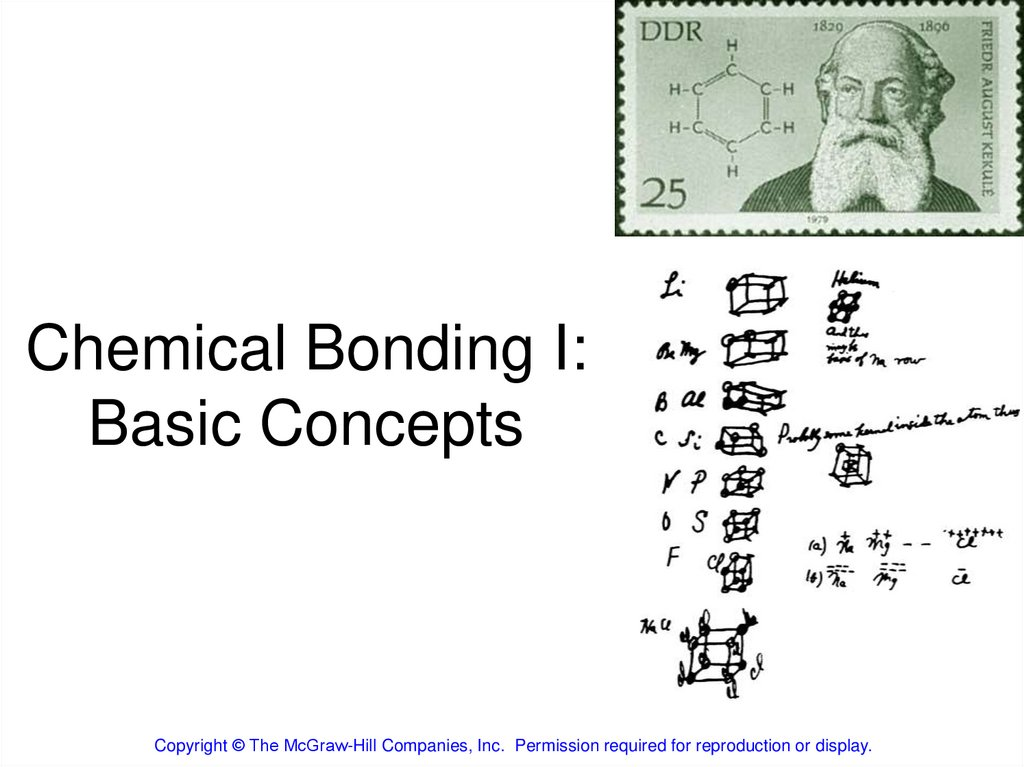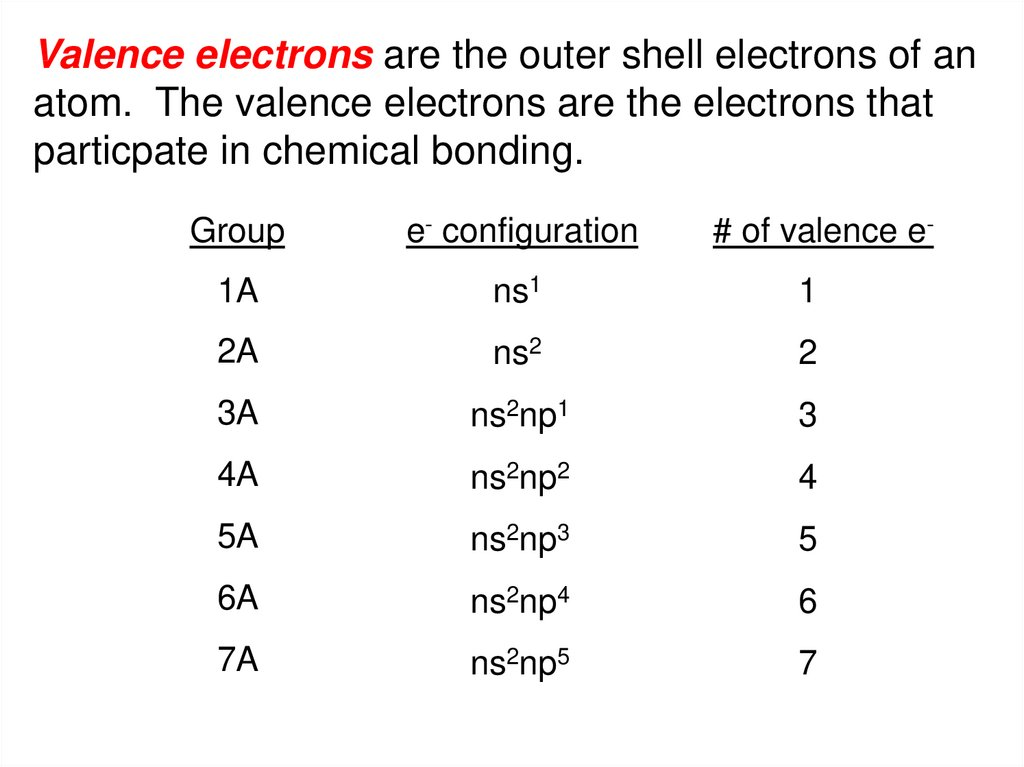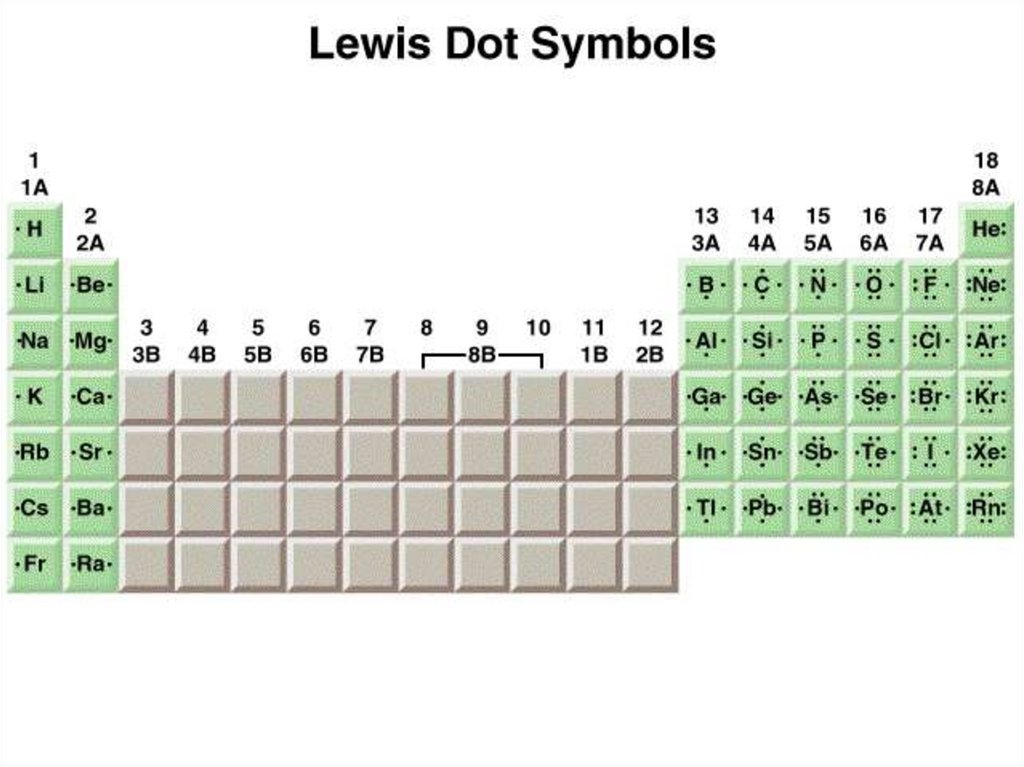# Chemical Bonding I: Basic Concepts

## 1. Chemical Bonding I: Basic Concepts

Copyright © The McGraw-Hill Companies, Inc. Permission required for reproduction or display.

## 2.

Valence electrons are the outer shell electrons of an
atom. The valence electrons are the electrons that
particpate in chemical bonding.
Group
e- configuration
# of valence e-
1A
ns1
1
2A
ns2
2
3A
ns2np1
3
4A
ns2np2
4
5A
ns2np3
5
6A
ns2np4
6
7A
ns2np5
7

The Ionic Bond
Li + F
1 22s22p5
1s22s1s
e- +
Li+ +
Li+ F [He]
1s
1s2[2Ne]
2s22p6
Li
Li+ + e-
F
F -
F -
Li+ F -

## 5.

A covalent bond is a chemical bond in which two or more
electrons are shared by two atoms.
Why should two atoms share electrons?
F
+
7e-
F
F F
7e-
8e- 8e-
Lewis structure of F2
single covalent bond
lone pairs
F
F
single covalent bond
lone pairs
F F
lone pairs
lone pairs

## 6.

Lewis structure of water
H
+
O +
H
single covalent bonds
H O H
or
H
O
H
2e-8e-2eDouble bond – two atoms share two pairs of electrons
O C O
or
O
O
C
double bonds
- 8e8e- 8ebonds
double
Triple bond – two atoms share three pairs of electrons
N N
triple
bond
8e-8e
or
N
N
triple bond

## 7.

Lengths of Covalent Bonds
Bond
Type
Bond
Length
(pm)
C-C
154
C C
133
C C
120
C-N
143
C N
138
C N
116
Bond Lengths
Triple bond < Double Bond < Single Bond

## 9.

Polar covalent bond or polar bond is a covalent
bond with greater electron density around one of the
two atoms
electron poor
region
H
electron rich
region
F
e- poor
H
d+
e- rich
F
d-

## 10.

Electronegativity is the ability of an atom to attract
toward itself the electrons in a chemical bond.
Electron Affinity - measurable, Cl is highest
X (g) + e-
X-(g)
Electronegativity - relative, F is highest

## 13.

Classification of bonds by difference in electronegativity
Difference
Bond Type
0
Covalent
2
0 < and <2
Ionic
Polar Covalent
Increasing difference in electronegativity
Covalent
Polar Covalent
share e-
partial transfer of e-
Ionic
transfer e-

## 14.

Classify the following bonds as ionic, polar covalent,
or covalent: The bond in CsCl; the bond in H2S; and
the NN bond in H2NNH2.
Cs – 0.7
Cl – 3.0
3.0 – 0.7 = 2.3
Ionic
H – 2.1
S – 2.5
2.5 – 2.1 = 0.4
Polar Covalent
N – 3.0
N – 3.0
3.0 – 3.0 = 0
Covalent

## 15.

Writing Lewis Structures
1. Draw skeletal structure of compound showing
what atoms are bonded to each other. Put least
electronegative element in the center.
2. Count total number of valence e-. Add 1 for
each negative charge. Subtract 1 for each
positive charge.
3. Complete an octet for all atoms except
hydrogen
4. If structure contains too many electrons, form
double and triple bonds on central atom as
needed.

## 16.

Write the Lewis structure of nitrogen trifluoride (NF3).
Step 1 – N is less electronegative than F, put N in center
Step 2 – Count valence electrons N - 5 (2s22p3) and F - 7 (2s22p5)
5 + (3 x 7) = 26 valence electrons
Step 3 – Draw single bonds between N and F atoms and complete
octets on N and F atoms.
Step 4 - Check, are # of e- in structure equal to number of valence e- ?
3 single bonds (3x2) + 10 lone pairs (10x2) = 26 valence electrons
F
N
F
F

## 17.

Write the Lewis structure of the carbonate ion (CO32-).
Step 1 – C is less electronegative than O, put C in center
Step 2 – Count valence electrons C - 4 (2s22p2) and O - 6 (2s22p4)
-2 charge – 2e4 + (3 x 6) + 2 = 24 valence electrons
Step 3 – Draw single bonds between C and O atoms and complete
octet on C and O atoms.
Step 4 - Check, are # of e- in structure equal to number of valence e- ?
3 single bonds (3x2) + 10 lone pairs (10x2) = 26 valence electrons
Step 5 - Too many electrons, form double bond and re-check # of e-
O
C
O
O
2 single bonds (2x2) = 4
1 double bond = 4
8 lone pairs (8x2) = 16
Total = 24

## 18.

Two possible skeletal structures of formaldehyde (CH2O)
H
C
O
H
H
C
H
O
An atom’s formal charge is the difference between the
number of valence electrons in an isolated atom and the
number of electrons assigned to that atom in a Lewis
structure.
formal charge
on an atom in
a Lewis
structure
=
total number
of valence
electrons in
the free atom
-
total number
of nonbonding
electrons
-
1
2
(
total number
of bonding
electrons
The sum of the formal charges of the atoms in a molecule
or ion must equal the charge on the molecule or ion.
)

## 19.

H
-1
+1
C
O
formal charge
on an atom in
a Lewis
structure
H
=
C – 4 eO – 6 e2H – 2x1 e12 e-
2 single bonds (2x2) = 4
1 double bond = 4
2 lone pairs (2x2) = 4
Total = 12
total number
total number
of valence
of nonbonding
electrons in electrons
the free atom
formal charge
= 4 -2 -½ x 6 = -1
on C
formal charge
= 6 -2 -½ x 6 = +1
on O
-
1
2
(
total number
of bonding
electrons
)

## 20.

H
H
0
C
formal charge
on an atom in
a Lewis
structure
0
O
=
C – 4 eO – 6 e2H – 2x1 e12 e-
2 single bonds (2x2) = 4
1 double bond = 4
2 lone pairs (2x2) = 4
Total = 12
total number
total number
of valence
of nonbonding
electrons in electrons
the free atom
formal charge
= 4 - 0 -½ x 8 = 0
on C
formal charge
= 6 -4 -½ x 4 = 0
on O
-
1
2
(
total number
of bonding
electrons
)

## 21.

Formal Charge and Lewis Structures
1. For neutral molecules, a Lewis structure in which there
are no formal charges is preferable to one in which
formal charges are present.
2. Lewis structures with large formal charges are less
plausible than those with small formal charges.
3. Among Lewis structures having similar distributions of
formal charges, the most plausible structure is the one in
which negative formal charges are placed on the more
electronegative atoms.
Which is the most likely Lewis structure for CH2O?
H
-1
+1
C
O
H
H
H
0
C
0
O